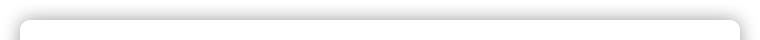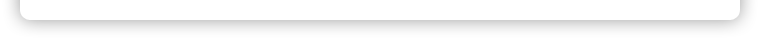# Order of Operations in JavaScript

Operators are evaluated in the order supplied in the following table. Associativity applies when more than one operation with the same precedence takes place. Left-to-right Associativity means that operations of equal weight in a single statement are performed from left to right.

Order Assoc Operator Summary
1 L
L
L
R
.
[]
()
new
Retrieve object property.
Array index or associative object property.
Function call.
Instantiate an object.
2 R
R
R
R
R
R
R
R
R
++
--
-
+
~
!
delete
typeof
void
Pre- or post-increment. (unary)
Pre- or post-decrement. (unary)
Negation. (unary, NOT subtraction)
No operation, use numerical value. (unary, NOT addition)
Bitwise complement. (unary)
Logical complement. (unary)
Destroy an object property. (unary)
Return datatype. (unary)
Return undefined. (unary)
3 L *, /, % Multiply, divide, modulo (remainder)
4 L
L
+, -
+
Concatenate strings.
5 L
L
L
<<
>>
>>>
Shift left.
Shift right and extend sign.
Shift right and pad with zeroes.
6 L
L
L
L
<, <=
>, >=
instanceof
in
Less than, less than or equal.
Greater than, greater than or equal.
Verify an object's constructor.
Verify an object's property exists.
7 L
L
L
L
==
!=
===
!==
Are operands equal?
Are operands NOT equal?
Are operands identical?
Are operands NOT identical?
8 L & Bitwise AND
9 L ^ Bitwise XOR
10 L | Bitwise OR
11 L && Local AND
12 L || Local OR
13 R ? : Conditional operator (ternary)
14 R
R
=
*=, /=, %=, +=, -=, <<=,
>>=, >>>=, &=, ^=, |=
Assign value.
Perform operation, then assign value.
15 L , (comma) Multiple evaluation.

var tags = [];